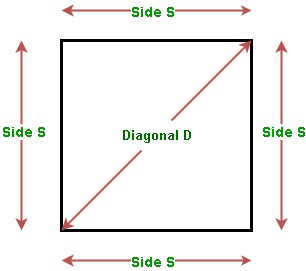# Area of a Square | Using Side, Diagonal and Perimeter

• Difficulty Level : Medium
• Last Updated : 07 Apr, 2021

Given one of the Sides S, Diagonal D, or Perimeter P of the square, the task is to find the area of the square with the given value.Attention reader! All those who say programming isn't for kids, just haven't met the right mentors yet. Join the  Demo Class for First Step to Coding Coursespecifically designed for students of class 8 to 12.

The students will get to learn more about the world of programming in these free classes which will definitely help them in making a wise career choice in the future.

Examples:

Input: S = 5
Output: Area of the square using side = 25

Input: D = 4
Output: Area of the square using diagonal = 8

Input: P = 32
Output: Area of the square using perimeter = 64

### Finding Area of Square using its Side

The area of the square of the side S is given by:

Area = Side * Side

Below is the implementation of the above approach:

## C++

 // C++ program for the above approach#include using namespace std; // Function to find the area of a squareint areaOfSquare(int S){    // Use above formula    int area = S * S;     return area;} // Driver Codeint main(){     // Given Side of square    int S = 5;     // Function call    cout << areaOfSquare(S);    return 0;}

## Java

 // Java program for the above approachclass GFG{  // Function to find the area of a squarestatic int areaOfSquare(int S){    // Use above formula    int area = S * S;      return area;}  // Driver Codepublic static void main(String[] args){      // Given Side of square    int S = 5;      // Function call    System.out.println(areaOfSquare(S));}} // This code is contributed by rock_cool

## Python3

 # Python3 program for the above approach # Function to find the area of a squaredef areaOfSquare(S):     # Use above formula    area = S * S     return area # Driver Codeif __name__ == '__main__':     # Given Side of square    S = 5     # Function call    print(areaOfSquare(S)) # This code is contributed by Mohit Kumar

## C#

 // C# program for the above approachusing System;class GFG{   // Function to find the area of a squarestatic int areaOfSquare(int S){    // Use above formula    int area = S * S;       return area;}   // Driver Codepublic static void Main(string[] args){       // Given Side of square    int S = 5;       // Function call    Console.Write(areaOfSquare(S));}} // This code is contributed by Ritik Bansal

## Javascript

 

Output:

25

### Finding Area of Square using its Diagonal

• The Area of the square of given side S is given by:

Area = S * S      …(1)

• The relation between Side S and Diagonal D is given by:…(2)

• Substituting the value of S from Equation (2) in Equation (1), we have:Below is the implementation of the above approach:

## C++

 // C++ program for the above approach#include using namespace std; // Function to find the area of a squareint areaOfSquare(int D){    // Use above formula    int area = (D * D) / 2;     return area;} // Driver Codeint main(){     // Given diagonal of square    int D = 4;     // Function call    cout << areaOfSquare(D);    return 0;}

## Java

 // Java program for the above approachimport java.util.*; class GFG{ // Function to find the area of a squarestatic int areaOfSquare(int D){         // Use above formula    int area = (D * D) / 2;     return area;} // Driver Codepublic static void main(String[] args){         // Given diagonal of square    int D = 4;     // Function call    System.out.print(areaOfSquare(D));}} // This code is contributed by PrinciRaj1992

## Python3

 # Python3 program for the above approach # Function to find the area of a squaredef areaOfSquare(D):     # Use above formula    area = (D * D) // 2;     return area; # Driver Codeif __name__ == '__main__':     # Given diagonal of square    D = 4;    # Function call    print(areaOfSquare(D)); # This code is contributed by PrinciRaj1992

## C#

 // C# program for the above approachusing System; class GFG{ // Function to find the area of a squarestatic int areaOfSquare(int D){         // Use above formula    int area = (D * D) / 2;     return area;} // Driver Codepublic static void Main(String[] args){         // Given diagonal of square    int D = 4;     // Function call    Console.Write(areaOfSquare(D));}} // This code is contributed by amal kumar choubey

## Javascript

 
Output:
8

### Finding Area of Square using its Perimeter

• The Area of the square of given side S is given by:

Area = S * S      …(1)

• The relation between Side S and Perimeter P is given by:

P =4*S      …(2)

• Substituting the value of S from Equation (2) in Equation (1), we have:Below is the implementation of the above formula:

## C++

 // C++ program for the above approach#include using namespace std; // Function to find the area of a squareint areaOfSquare(int P){    // Use above formula    int area = (P * P) / 16;     return area;} // Driver Codeint main(){    // Given perimeter of square    int P = 32;     // Function call    cout << areaOfSquare(P);    return 0;}

## Java

 // Java program for the above approachclass GFG{ // Function to find the area of a squarestatic int areaOfSquare(int P){         // Use above formula    int area = (P * P) / 16;     return area;} // Driver Codepublic static void main(String[] args){         // Given perimeter of square    int P = 32;     // Function call    System.out.print(areaOfSquare(P));}} // This code is contributed by amal kumar choubey

## Python3

 # Python3 program for the above approach # Function to find the area of a squaredef areaOfSquare(P):       # Use above formula    area = (P * P) // 16;     return area; # Driver Codeif __name__ == '__main__':       # Given perimeter of square    P = 32;     # Function call    print(areaOfSquare(P)); # This code is contributed by gauravrajput1

## C#

 // C# program for the above approachusing System; class GFG{ // Function to find the area of a squarestatic int areaOfSquare(int P){         // Use above formula    int area = (P * P) / 16;     return area;} // Driver Codepublic static void Main(String[] args){         // Given perimeter of square    int P = 32;     // Function call    Console.Write(areaOfSquare(P));}} // This code is contributed by amal kumar choubey

## Javascript

 
Output:
64

My Personal Notes arrow_drop_up